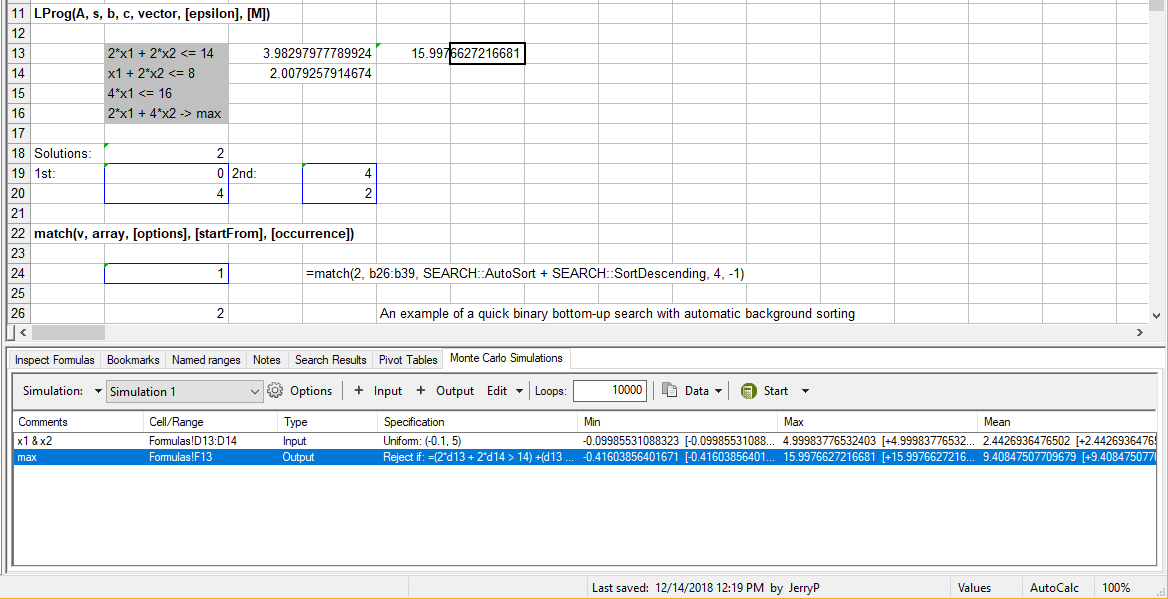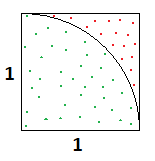Using Monte Carlo Simulations

The Monte Carlo method can be used to analyze data with help of random number generators. This includes for example finding approximate maximum and minimum for a given formula/function or simulating real data like measurements or observations.

In GS-Calc the Monte Carlo simulations are executed by specifying "Input" worksheet cells or ranges which are filled with ("pseudo-") random numbers in loops and "Output" cells which contain formulas processing these numbers. Large numbers of loops enable collecting statistically meaningful number of results of those formulas and verifying how they will behave for real "Input" data.

The following menus and commands related to the MC simulations are available in GS-Calc:

• Simulations
• Adding new simulations with input and output cells and optionally specifying random number generator parameters. (See: the specification of the mtxRand functions).
• Adding a copy of an existing simulation.
• Deleting the selected simulation or all simulations.
• Add Input/Output cells or ranges

Added input worksheet cells should be empty or should contain only numbers. They are filled with random numbers in subsequent loops.
Added output cells should contain formulas processing these numbers and returning numeric values.

Output cells can use a "rejection" formula which determines whether the results obtained in a given loop should be retained and included in the calculated statistics.

Example (I): to find an approx. solution of the linear programming problem:2*x1 + 2*x2 <= 14
x1 + 2*x2 <= 8
4*x1 <= 16
2*x1 + 4*x2 -< max

If you specify D13 and D14 as input cells with the uniform distribution (-0.1, 5) and E13 as the output cell with the formula:

=2*d13 + 4*d14

with the following rejection criteria:

=(2*d13 + 2*d14 > 14) + (d13 + 2*d14 > 8) + (4*d13 > 16)

Then after 10000 loops (and for the default generator parameters), the found maximum of E13 will be around 15.997 for the x1=3.983 and x2=2.01 (the exact values calculated with the LProg() function are respectively 16, 4 and 2).

Note:The same output cells can be added many times so the above constrains doesn't have to be just one merged rejection criteria.

Example (II): to calculate the area of a cirle with a radius r=1:

Specify B2 and B3 as the input cells with the uniform distribution (0, 1) and D2 as the output cell with the formula:

=sqrt(b2*b2 + b3*b3)

with the following rejection criteria:

=sqrt(b2*b2 + b3*b3) > 1Then after 1000000 loops (and for the default generator parameters), the "counter" value for the (not rejected) output cell will be 785348. Thus the approx. area of the 1/4th of that cirle is 785348/1000000 and the full area is approx. equal to 3.141392 which is the approximation of the real value of Pi*r^2 = 3.14159265358979.

• Showing min, max
Selecting a desirable item on the "Input"/"Output" list and choosing the "Data ->Show Min/Max" commands will fill the input/output worksheet cells with the loop input/output data where this minimum or maximum was found.
• Saving generated loop data
After performing the specified number of loops the data are ready to be saved. They are stored as a new worksheet in the specified folder. Loops are saved in rows so if the number of the selected input/output cells exceeds the maximum number of columns (4096), the saved data will be truncated.
Input/output items specified as ranges are expanded (likewise, in rows) in that new worksheet as individual cells.
• Starting simulation, cancelling, resuming

Executing loops can stopped and resumed at any moment.

During the procedure the list of the input/output cells is updated every 0.5s to display the current:
maximum
minimum
mean
variance
standard deviation
counter (for output cells this excludes rejected loops).

Values displayed in the [] square brackets denote the change in comparison with the previous screen update (0.5s).

Starting the simulation stops any pending workbook updates and vice-versa.